# Shooters

In army regiment are six shooters. The first shooter target hit with a probability of 49%, next with 75%, 41%, 20%, 34%, 63%. Calculate the probability of target hit when shooting all at once.

p =  98.53 %

### Step-by-step explanation: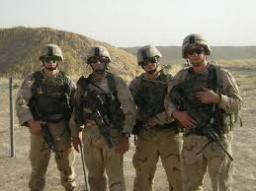Did you find an error or inaccuracy? Feel free to write us. Thank you!Tips to related online calculators
Would you like to compute count of combinations?

## Related math problems and questions:

• Three shooters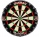Three shooters shoot, each one time, on the same target. The first hit the target with a probability of 0.7; second with a probability of 0.8 and a third with a probability of 0.9. What is the probability to hit the target: a) just once b) at least once c
• The rawThe raw data presented here are the scores (out of 100 marks) of a market survey regarding the acceptability of new product launched by a company for a random sample of 50 respondents: 40 45 41 45 45 30 30 8 48 25 26 9 23 24 26 29 8 40 41 42 39 35 18 25 3
• Shooter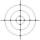The probability that a good shooter hits the center of the target circle I is 0.1. The probability that the target hit the inner circle II is 0.58. What is the probability that it hits the target circle I or II?
• Box and whisker plotConstruct a box and whisker plot for the given data. 56, 32, 54, 32, 23, 67, 23, 45, 12, 32, 34, 24, 36, 47, 19, 43
• Standard deviationFind standard deviation for dataset (grouped data): Age (years) No. Of Persons 0-10 15 10-20 15 20-30 23 30-40 22 40-50 25 50-60 10 60-70 5 70-80 10
• N percentille problemHere is a data set (n=117) that has been sorted. 10.4 12.2 14.3 15.3 17.1 17.8 18 18.6 19.1 19.9 19.9 20.3 20.6 20.7 20.7 21.2 21.3 22 22.1 22.3 22.8 23 23 23.1 23.5 24.1 24.1 24.4 24.5 24.8 24.9 25.4 25.4 25.5 25.7 25.9 26 26.1 26.2 26.7 26.8 27.5 27.6 2
• TrianglesFind out whether given sizes of the angles can be interior angles of a triangle: a) 23°10',84°30',72°20' b) 90°,41°33',48°37' c) 14°51',90°,75°49' d) 58°58',59°59',60°3'
• Alcohol 2Two types of alcohol one 63% and second 75% give 20 liters of 69% alcohol. How many liters of each type are in the mixture?
• The shooter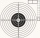The shooter shoots at the target, assuming that the individual shots are independent of each other and the probability of hitting each of them is 0.2. The shooter fires until he hits the target for the first time, then stop firing. (a) What is the most li
• The manufacturer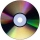The manufacturer found that 3% of the plates produced had a malfunction. Of the compliant, 75% are first and 25% second. What is the probability of producing first and second class plates?
• Utopia IslandThe probability of disease A on the island of Utopia is 40%. The probability of occurrence among the men of this island, which make up 60% of all the population (the rest are women), is 50%. What is the probability of occurrence of A disease among women o
• Speed of Slovakian trainsRudolf decided to take the train from the station 'Ostratice' to 'Horné Ozorovce'. In the train timetables found train Os 5409 : km 0 Chynorany 15:17 5 Ostratice 15:23 15:23 8 Rybany 15:27 15:27 10 Dolné Naštice 15:31 15:31 14 Bánovce nad Bebravou 15:35 1
• Sum of fall dices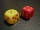What is the probability that the sum of 9 will fall on a roll of two dice? Hint: write down all the pairs that can occur as follows: 11 12 13 14 15. . 21 22 23 24. .. . 31 32. .. . . . . . .. . 66, count them, it's the variable n variable m: 36, 63,. .. .
• Reverse Pythagorean theoremGiven are lengths of the sides of the triangles. Decide which one is rectangular: Δ ABC: 77 dm, 85 dm, 36 dm ... Δ DEF: 55 dm, 82 dm, 61 dm ... Δ GHI: 24 mm, 25 mm, 7 mm ... Δ JKL: 32 dm, 51 dm, 82 dm ... Δ MNO: 51 dm, 45 dm,
• RemaindersIt is given a set of numbers { 170; 244; 299; 333; 351; 391; 423; 644 }. Divide this numbers by number 66 and determine set of remainders. As result write sum of this remainders.
• Bulbs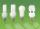In the box are 6 bulbs with power 75 W, 14 bulbs with power 40 W and 15 with 60 W. Calculate the probability that a randomly selected bulb is:
• The shooter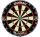The shooter heard the impact of the bullet on the target in one second after the shot. The bullet was moving at an average speed of 500 m/s. Calculate with speed of sound of 340 m/s. Determine the distance of the target.